# 6 Figure Grid References It is useful to

• Slides: 16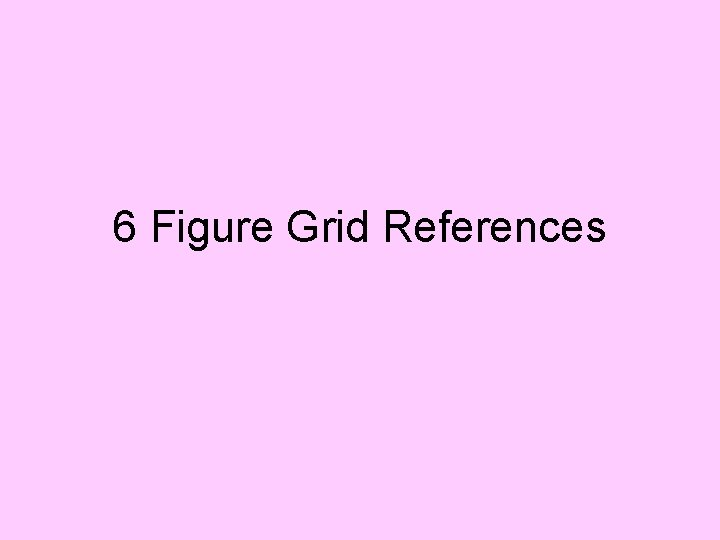6 Figure Grid References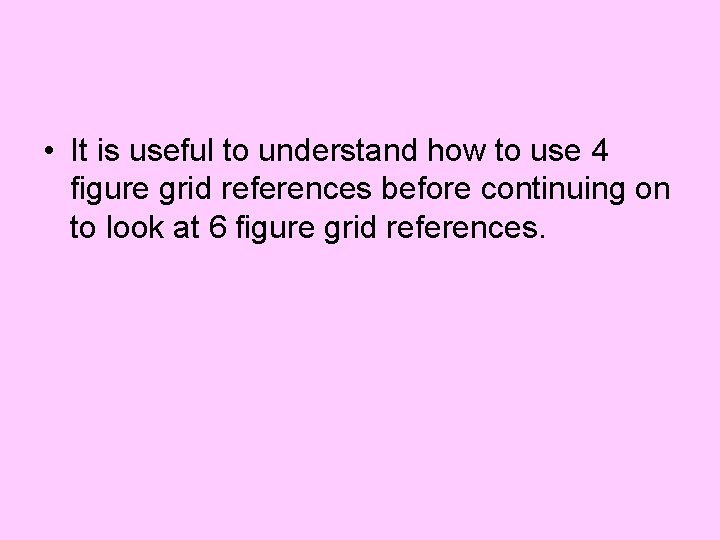• It is useful to understand how to use 4 figure grid references before continuing on to look at 6 figure grid references.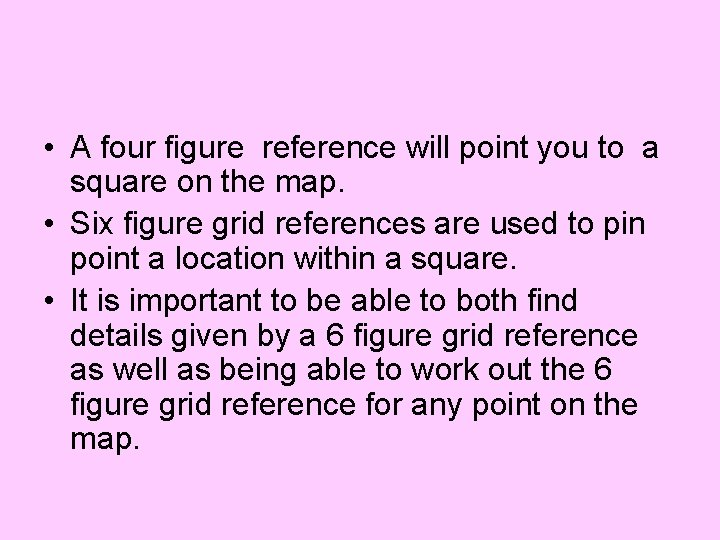• A four figure reference will point you to a square on the map. • Six figure grid references are used to pin point a location within a square. • It is important to be able to both find details given by a 6 figure grid reference as well as being able to work out the 6 figure grid reference for any point on the map.How to work out 6 figure grid references… • Let us first look at how the 6 figure grid reference is made up. • Let us take 216 322 as an example. • The first 3 figures refer to the easting of the point (or the distance along the map) • While the second 3 figures refer to the northing of the point (or the distance up the map).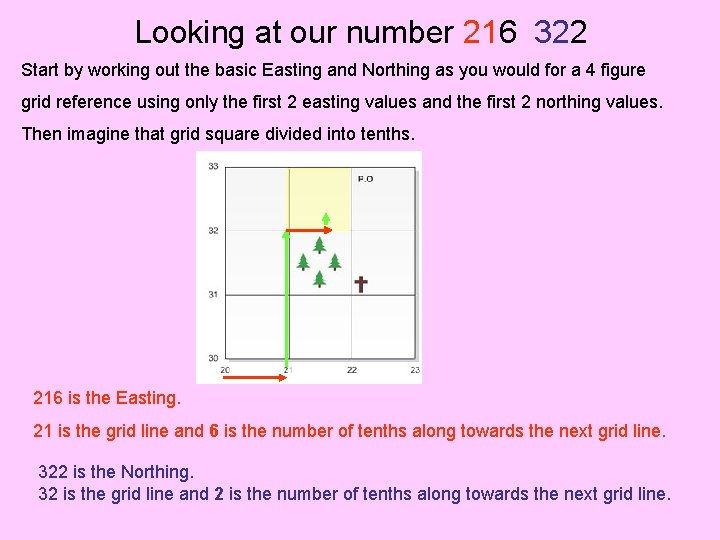Looking at our number 216 322 Start by working out the basic Easting and Northing as you would for a 4 figure grid reference using only the first 2 easting values and the first 2 northing values. Then imagine that grid square divided into tenths. 216 is the Easting. 21 is the grid line and 6 is the number of tenths along towards the next grid line. 322 is the Northing. 32 is the grid line and 2 is the number of tenths along towards the next grid line.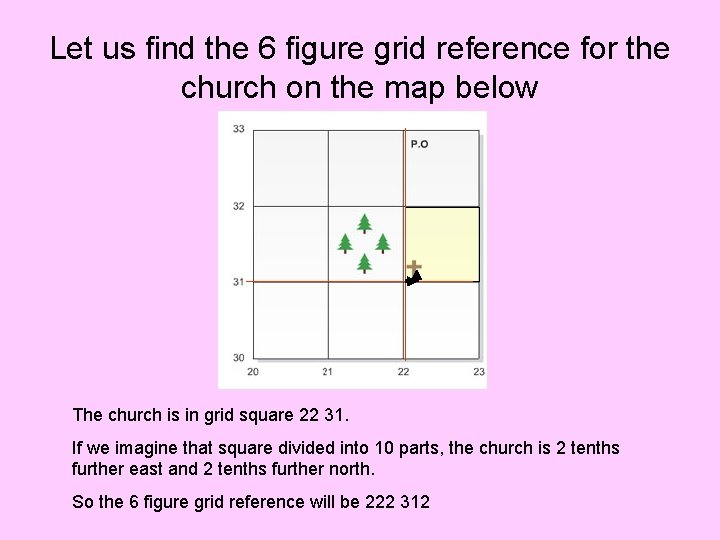Let us find the 6 figure grid reference for the church on the map below The church is in grid square 22 31. If we imagine that square divided into 10 parts, the church is 2 tenths further east and 2 tenths further north. So the 6 figure grid reference will be 222 312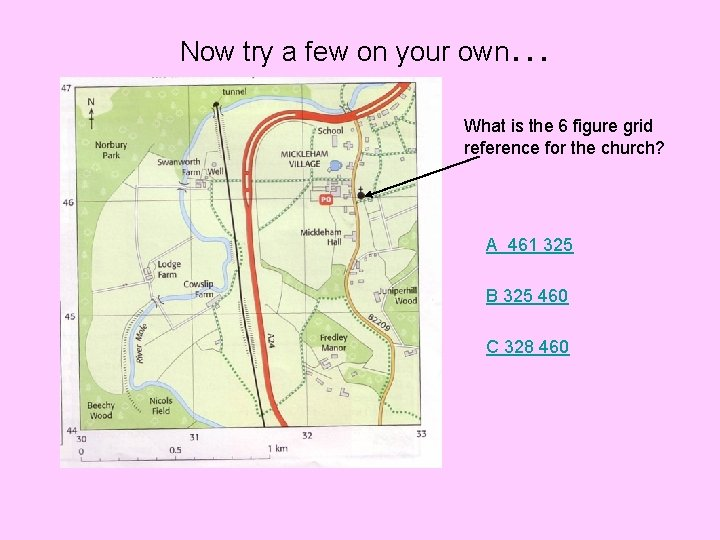Now try a few on your own… What is the 6 figure grid reference for the church? A 461 325 B 325 460 C 328 460• The answer is 328 460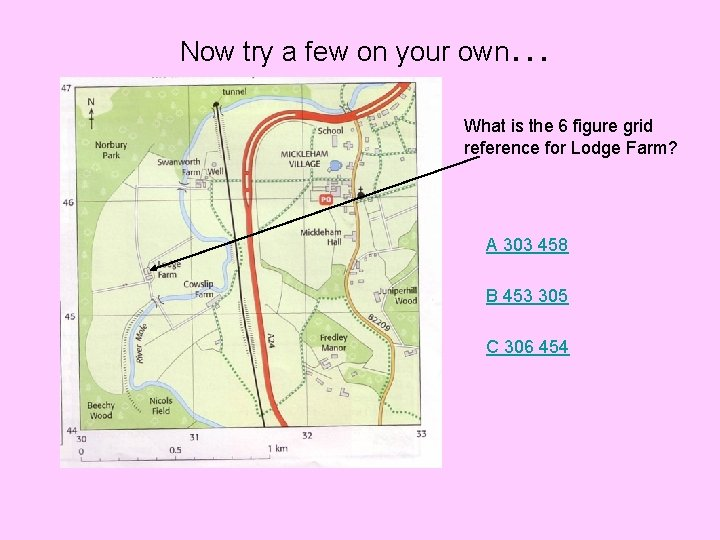Now try a few on your own… What is the 6 figure grid reference for Lodge Farm? A 303 458 B 453 305 C 306 454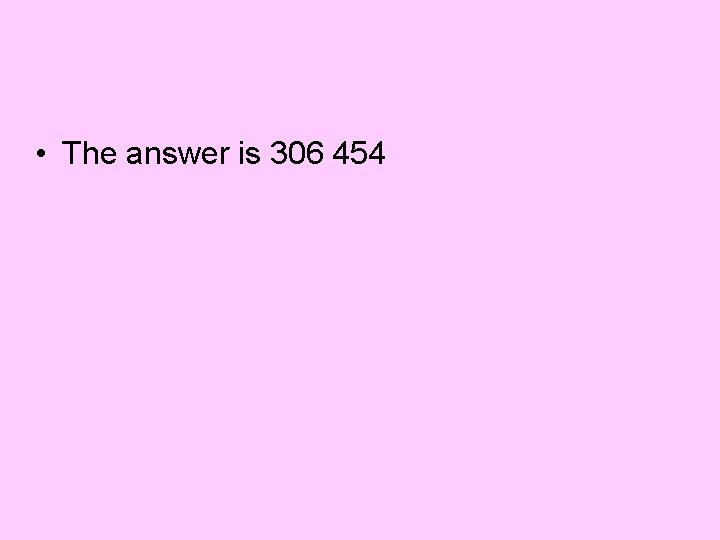• The answer is 306 454Now try a few on your own… What is the 6 figure grid reference for the school? A 323 465 B 332 456 C 313 465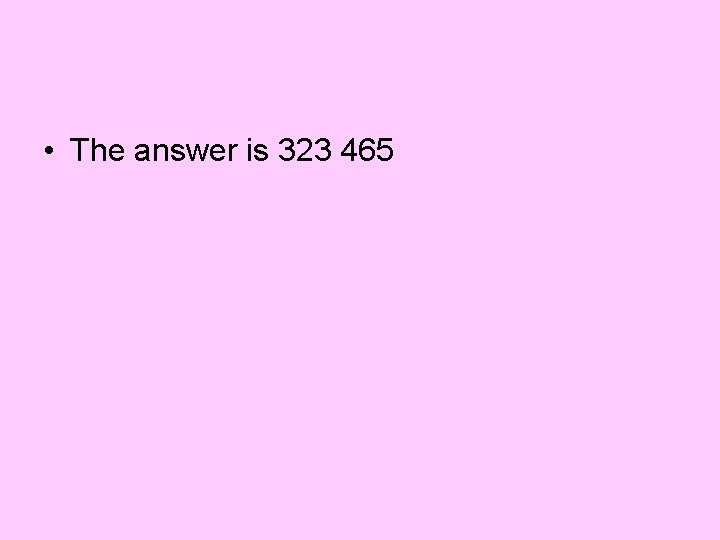• The answer is 323 465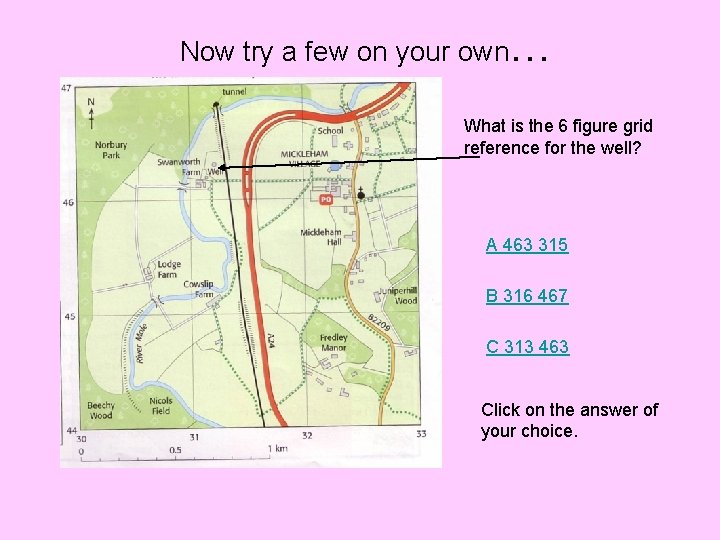Now try a few on your own… What is the 6 figure grid reference for the well? A 463 315 B 316 467 C 313 463 Click on the answer of your choice.• The answer is 313 463Now try a few on your own… What is at 6 figure grid reference 325 456? A The Post Office B Mickleham Hall C Cowslip Farm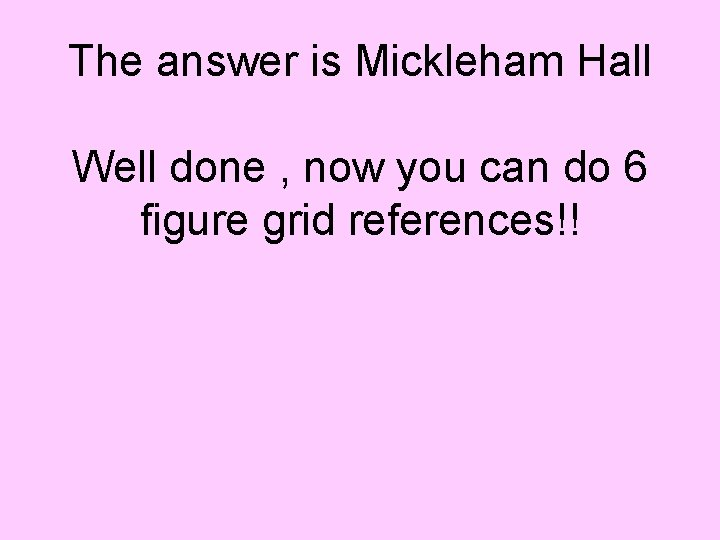The answer is Mickleham Hall Well done , now you can do 6 figure grid references!!